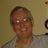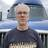### I’ve got this code from a button on a form but get error “not a data field” on the last line. Any help appreciated.let DD := i.'Next One';
while DD <= today() do
let DD := DD+28
end
i.'Next One' := DDIs "i" a table reference?Sorry, yes it is (Let i:= select Transactions)`let i:= select Transactions`

is going to return an array of Id's for the Transactions table because Id is the default if you don't specfy another field like...

`let i := (select Transactions).'Next One'`

So, you have "i" which is an array. How do you determine which element in the array is being referenced? Once you determine that, if you want to use the array to represent the record in the table you would have to use a format like this...

`item(i, N).'Next One' := DD`

Keep in mind arrays are 0 based.Thanks Sean, I’ll give it a go.Lawrie, if you are just trying to access the current record use something like this...

`let DD := this.'Next One';`
`        while DD <= today() do`
`            let DD := DD+28`
`        end`
`this.'Next One' := DD`👍